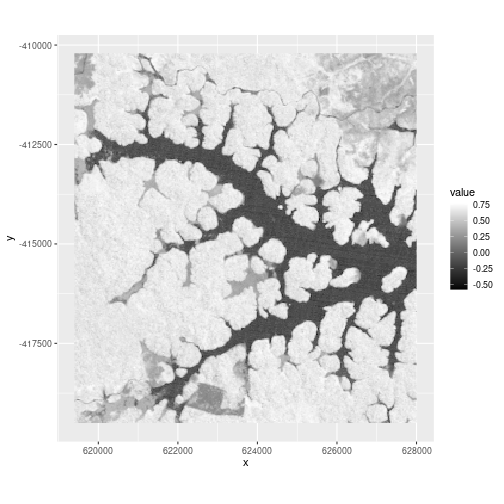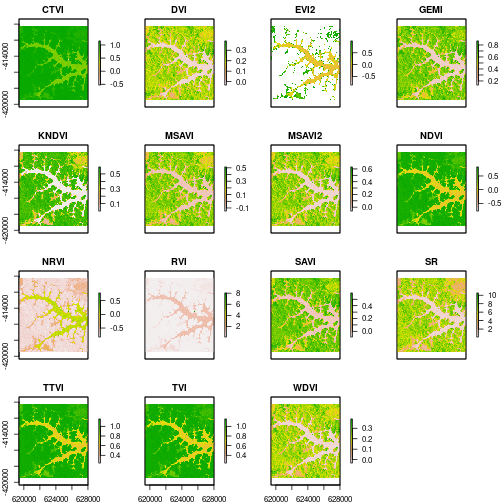spectralIndices { RStoolbox} R Documentation

### Spectral Indices

R: Spectral Indices

### Description

Calculate a suite of multispectral indices such as NDVI, SAVI etc. in an efficient way.

### Usage

```spectralIndices(
img,
blue = NULL,
green = NULL,
red = NULL,
nir = NULL,
redEdge1 = NULL,
redEdge2 = NULL,
redEdge3 = NULL,
swir1 = NULL,
swir2 = NULL,
swir3 = NULL,
scaleFactor = 1,
skipRefCheck = FALSE,
indices = NULL,
index = NULL,
coefs = list(L = 0.5, G = 2.5, L_evi = 1, C1 = 6, C2 = 7.5, s = 1, swir2ccc = NULL,
swir2coc = NULL),
...
)
```

### Arguments

 `img` Raster* object. Typically remote sensing imagery, which is to be classified. `blue` Character or integer. Blue band. `green` Character or integer. Green band. `red` Character or integer. Red band. `nir` Character or integer. Near-infrared band (700-1100nm). `redEdge1` Character or integer. Red-edge band (705nm) `redEdge2` Character or integer. Red-edge band (740nm) `redEdge3` Character or integer. Red-edge band (783nm) `swir1` not used `swir2` Character or integer. Short-wave-infrared band (1400-1800nm). `swir3` Character or integer. Short-wave-infrared band (2000-2500nm). `scaleFactor` Numeric. Scale factor for the conversion of scaled reflectances to [0,1] value range (applied as reflectance/scaleFactor) Neccesary for calculating EVI/EVI2 with scaled reflectance values. `skipRefCheck` Logical. When EVI/EVI2 is to be calculated there is a rough heuristic check, whether the data are inside [0,1]+/-0.5 (after applying a potential `scaleFactor`). If there are invalid reflectances, e.g. clouds with reflectance > 1 this check will result in a false positive and skip EVI calculation. Use this argument to skip this check in such cases *iff* you are sure the data and scaleFactor are valid. `indices` Character. One or more spectral indices to calculate (see Details). By default (NULL) all implemented indices given the spectral bands which are provided will be calculated. `index` Character. Alias for `indices`. `maskLayer` RasterLayer containing a mask, e.g. clouds, for which pixels are set to NA. Alternatively a layername or -number can be provided if the mask is part of `img`. `maskValue` Integer. Pixel value in `maskLayer` which should be masked in output, i.e. will be set to `NA` in all calculated indices. `coefs` List of coefficients (see Details). `...` further arguments such as filename etc. passed to writeRaster

### Details

`spectralIndices` calculates all indices in one go in C++, which is more efficient than calculating each index separately (for large rasters). By default all indices which can be calculated given the specified indices will be calculated. If you don't want all indices, use the `indices` argument to specify exactly which indices are to be calculated. See the table bellow for index names and required bands.

Index values outside the valid value ranges (if such a range exists) will be set to NA. For example a pixel with NDVI > 1 will be set to NA.

 Index Description Source Bands Formula CLG Green-band Chlorophyll Index Gitelson2003 `redEdge3, green` redEdge3/green - 1 CLRE Red-edge-band Chlorophyll Index Gitelson2003 `redEdge3, redEdge1` redEdge3/redEdge1 - 1 CTVI Corrected Transformed Vegetation Index Perry1984 `red, nir` (NDVI + 0.5)/sqrt(abs(NDVI + 0.5)) DVI Difference Vegetation Index Richardson1977 `red, nir` s * nir - red EVI Enhanced Vegetation Index Huete1999 `red, nir, blue` G * ((nir - red)/(nir + C1 * red - C2 * blue + L_evi)) EVI2 Two-band Enhanced Vegetation Index Jiang 2008 `red, nir` G * (nir - red)/(nir + 2.4 * red + 1) GEMI Global Environmental Monitoring Index Pinty1992 `red, nir` (((nir^2 - red^2) * 2 + (nir * 1.5) + (red * 0.5))/(nir + red + 0.5)) * (1 - ((((nir^2 - red^2) * 2 + (nir * 1.5) + (red * 0.5))/(nir + red + 0.5)) * 0.25)) - ((red - 0.125)/(1 - red)) GNDVI Green Normalised Difference Vegetation Index Gitelson1998 `green, nir` (nir - green)/(nir + green) KNDVI Kernel Normalised Difference Vegetation Index Camps-Valls2021 `red, nir` tanh(((nir - red)/(nir + red)))^2 MCARI Modified Chlorophyll Absorption Ratio Index Daughtery2000 `green, red, redEdge1` ((redEdge1 - red) - (redEdge1 - green)) * (redEdge1/red) MNDWI Modified Normalised Difference Water Index Xu2006 `green, swir2` (green - swir2)/(green + swir2) MSAVI Modified Soil Adjusted Vegetation Index Qi1994 `red, nir` nir + 0.5 - (0.5 * sqrt((2 * nir + 1)^2 - 8 * (nir - (2 * red)))) MSAVI2 Modified Soil Adjusted Vegetation Index 2 Qi1994 `red, nir` (2 * (nir + 1) - sqrt((2 * nir + 1)^2 - 8 * (nir - red)))/2 MTCI MERIS Terrestrial Chlorophyll Index DashAndCurran2004 `red, redEdge1, redEdge2` (redEdge2 - redEdge1)/(redEdge1 - red) NBRI Normalised Burn Ratio Index Garcia1991 `nir, swir3` (nir - swir3)/(nir + swir3) NDREI1 Normalised Difference Red Edge Index 1 GitelsonAndMerzlyak1994 `redEdge2, redEdge1` (redEdge2 - redEdge1)/(redEdge2 + redEdge1) NDREI2 Normalised Difference Red Edge Index 2 Barnes2000 `redEdge3, redEdge1` (redEdge3 - redEdge1)/(redEdge3 + redEdge1) NDVI Normalised Difference Vegetation Index Rouse1974 `red, nir` (nir - red)/(nir + red) NDVIC Corrected Normalised Difference Vegetation Index Nemani1993 `red, nir, swir2` (nir - red)/(nir + red) * (1 - ((swir2 - swir2ccc)/(swir2coc - swir2ccc))) NDWI Normalised Difference Water Index McFeeters1996 `green, nir` (green - nir)/(green + nir) NDWI2 Normalised Difference Water Index Gao1996 `nir, swir2` (nir - swir2)/(nir + swir2) NRVI Normalised Ratio Vegetation Index Baret1991 `red, nir` (red/nir - 1)/(red/nir + 1) REIP Red Edge Inflection Point GuyotAndBarnet1988 `red, redEdge1, redEdge2, redEdge3` 0.705 + 0.35 * ((red + redEdge3)/(2 - redEdge1))/(redEdge2 - redEdge1) RVI Ratio Vegetation Index `red, nir` red/nir SATVI Soil Adjusted Total Vegetation Index Marsett2006 `red, swir2, swir3` (swir2 - red)/(swir2 + red + L) * (1 + L) - (swir3/2) SAVI Soil Adjusted Vegetation Index Huete1988 `red, nir` (nir - red) * (1 + L)/(nir + red + L) SLAVI Specific Leaf Area Vegetation Index Lymburger2000 `red, nir, swir2` nir/(red + swir2) SR Simple Ratio Vegetation Index Birth1968 `red, nir` nir/red TTVI Thiam's Transformed Vegetation Index Thiam1997 `red, nir` sqrt(abs((nir - red)/(nir + red) + 0.5)) TVI Transformed Vegetation Index Deering1975 `red, nir` sqrt((nir - red)/(nir + red) + 0.5) WDVI Weighted Difference Vegetation Index Richardson1977 `red, nir` nir - s * red

Some indices require additional parameters, such as the slope of the soil line which are specified via a list to the `coefs` argument. Although the defaults are sensible values, values like the soil brightnes factor `L` for SAVI should be adapted depending on the characteristics of the scene. The coefficients are:

 Coefficient Description Affected Indices `s` slope of the soil line DVI, WDVI `L_evi, C1, C2, G` various EVI `L` soil brightness factor SAVI, SATVI `swir2ccc` minimum swir2 value (completely closed forest canopy) NDVIC `swir2coc` maximum swir2 value (completely open canopy) NDVIC

The wavelength band names are defined following Schowengertd 2007, p10. The last column shows exemplarily which Landsat 5 TM bands correspond to which wavelength range definition.

 Band Description Wavl_min Wavl_max Landsat5_Band Sentinel2_Band vis visible 400 680 1,2,3 2,3,4 red-edge1 red-edge1 680 720 - 5 red-edge2 red-edge2 720 760 - 6 red-edge3 red-edge3 760 800 - 7 nir near infra-red 800 1100 4 8/8a swir1 short-wave infra-red 1100 1351 - 9,10 swir2 short-wave infra-red 1400 1800 5 11 swir3 short-wave infra-red 2000 2500 7 12 mir1 mid-wave infra-red 3000 4000 - - mir2 mid-wave infra-red 45000 5000 - - tir1 thermal infra-red 8000 9500 - - tir2 thermal infra-red 10000 140000 6 -

### Value

RasterBrick or a RasterLayer if length(indices) == 1

### Examples

``````library(ggplot2)
library(raster)
data(lsat)

## Calculate NDVI
ndvi <- spectralIndices(lsat, red = "B3_dn", nir = "B4_dn", indices = "NDVI")
ndvi
``````
``````#> class      : RasterLayer
#> dimensions : 310, 287, 88970  (nrow, ncol, ncell)
#> resolution : 30, 30  (x, y)
#> extent     : 619395, 628005, -419505, -410205  (xmin, xmax, ymin, ymax)
#> crs        : +proj=utm +zone=22 +datum=WGS84 +units=m +no_defs +ellps=WGS84 +towgs84=0,0,0
#> source     : memory
#> names      : NDVI
#> values     : -0.5789474, 0.762963  (min, max)
``````
``````ggR(ndvi, geom_raster = TRUE) +
````````````## No test:

## Calculate all possible indices, given the provided bands
## Convert DNs to reflectance (required to calculate EVI and EVI2)
mtlFile  <- system.file("external/landsat/LT52240631988227CUB02_MTL.txt", package="RStoolbox")
lsat_ref <- radCor(lsat, mtlFile, method = "apref")

SI <- spectralIndices(lsat_ref, red = "B3_tre", nir = "B4_tre")
plot(SI)
````````````## End(No test)
``````

[Package RStoolbox version 0.3.0 Index]